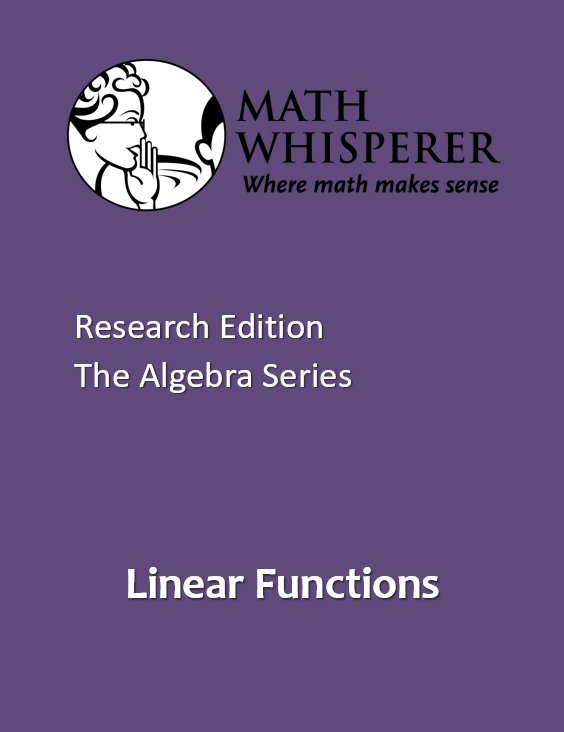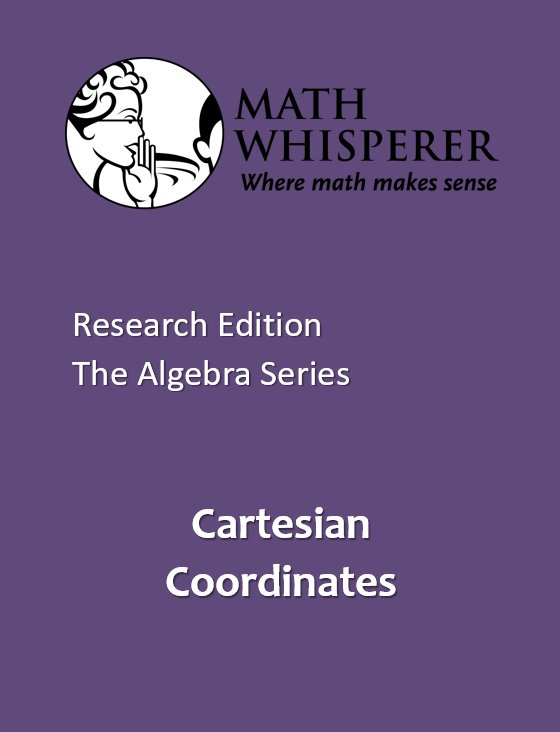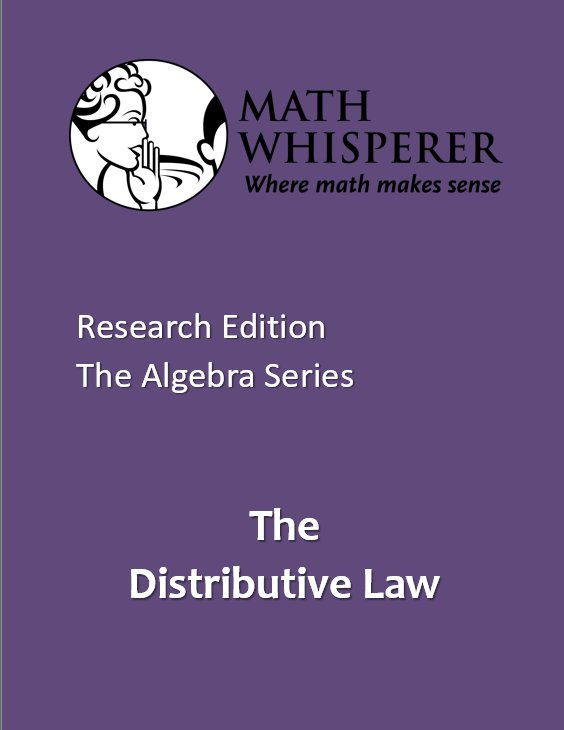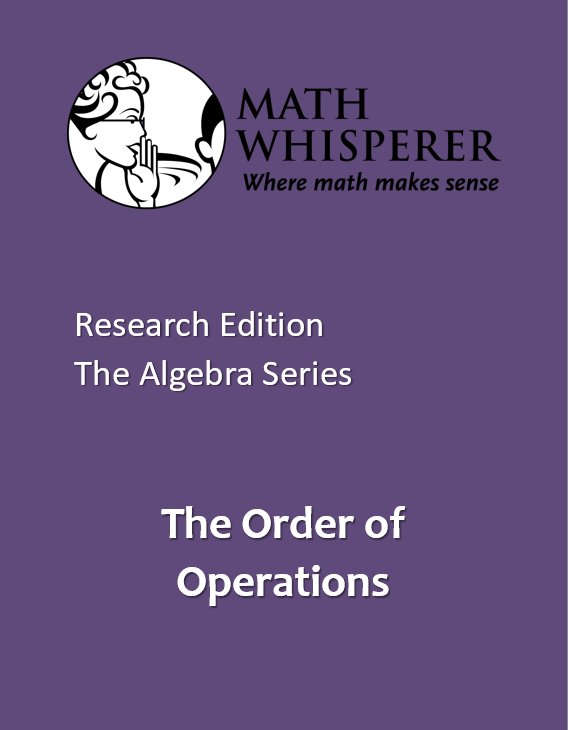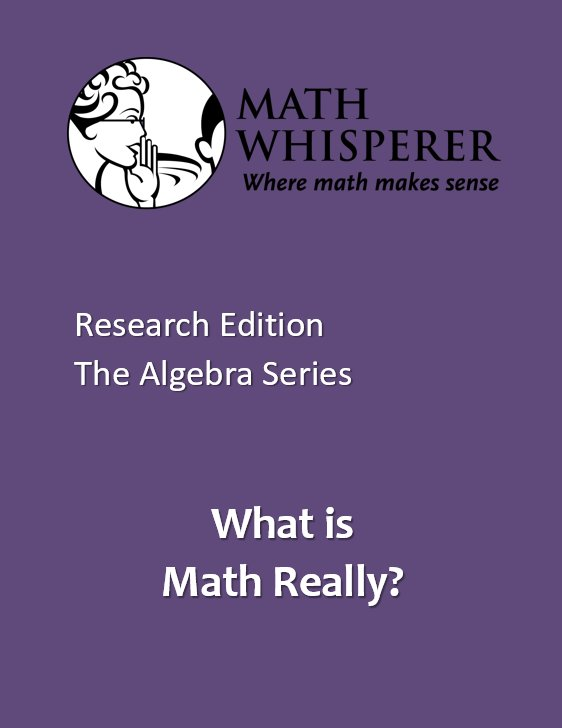Select Page

# The Algebra Series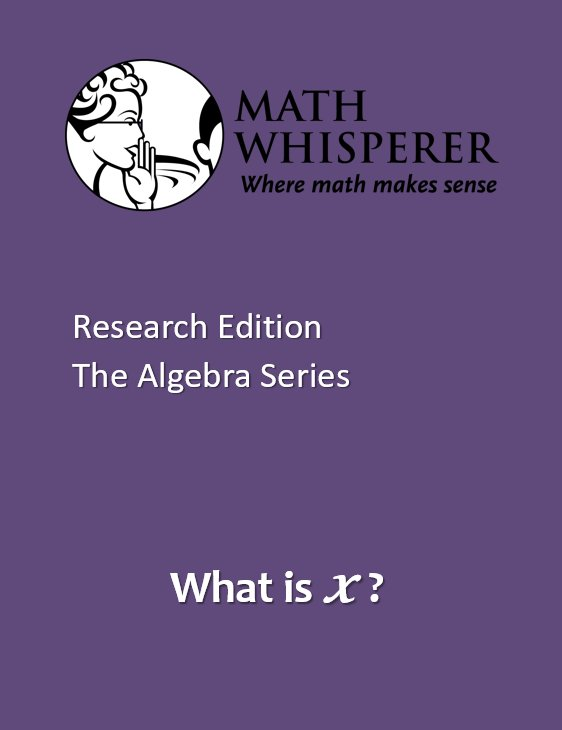The learning objectives in each of the lessons are these: What is x ?: The abstract concept of variable is introduced.Substitution: The idea that a number can be exchanged for a variable is introduced. Showing that x + x = 2 : Algebraic expressions like “2 ” make complete sense to those of us who have done algebra for years. But understanding that an expression like this means “2 multiplied by ” is not always obvious. Solving for x: This is where students solve the first set of actual problems. Actually solving reasonably complicated equations with reliability and confidence feels good!

Functions: These exercises/problems build confidence in the principles in the prior lessons. And problems like these appear on all sorts of state and other tests—now your student has a chance to solve them, when he or she may not have been able to do so before. But that is not the goal of this lesson, just a positive consequence.

Grade 6 and above: “x” is the world’s most popular variable in Algebra. For many students, “x” is confusing. This book makes “x” make sense, so that students can succeed in Algebra.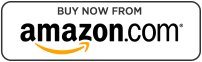## Coming soon